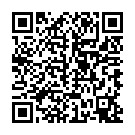## Missing Digits Multiplication

### Related Worksheets

Find the missing digit in a calculation. Choose addition, subtraction, multiplication or division. Lots of control over level inculding decimals.Scan to open this game on a mobile device. Right-click to copy and paste it onto a homework sheet.

### Game Objectives

New Maths Curriculum:

Year 4: Multiply two-digit and three-digit numbers by a one-digit number using formal written layout

Year 5: Multiply numbers up to 4 digits by a one- or two-digit number using an efficient written method, including long multiplication for two-digit numbers

Year 6: Multiply multi-digit numbers up to 4 digits by a two-digit whole number using the efficient written method of long multiplication

KS2 Primary Framework:

Year 3:

Use practical and informal written methods to multiply and divide two–digit numbers. (Block A)

Develop and use written methods to record, support or explain addition of two–digit and three–digit numbers (Block A)

Year 4:

Develop and use written methods to record, support and explain multiplication and division of two-digit numbers by a one-digit number. (Block A and Block D)

Refine and use efficient written methods to add two-digit and three-digit whole numbers. (Block A)

Derive and recall multiplication facts up to 10 × 10, the corresponding division facts (Block B and E)

Year 5:

Refine and use efficient written methods to multiply and divide HTU × U, TU × TU, U.t × U and HTU ÷ U (Block A and Block D and Block E)

Extend mental methods for whole-number calculations, for example to multiply a two-digit by a one-digit number (e.g. 12 × 9), to multiply by 25 (e.g. 16 × 25). (Block A)

Use efficient written methods to add and subtract whole numbers and decimals with up to two places (Block A and Block B)

Year 6:

Calculate mentally with integers and decimals: U.t ± U.t, TU × U, TU ÷ U, U.t × U, U.t ÷ U (Block A)

Use efficient written methods to add and subtract integers and decimals, to multiply and divide integers and decimals by a one-digit integer, and to multiply two-digit and three-digit integers by a two-digit integer (Block A, Block B, Block D and Block E)

Use knowledge of place value and multiplication facts to 10 × 10 to derive related multiplication and division facts involving decimals (e.g. 0.8 × 7, 4.8 ÷ 6) (Block B)